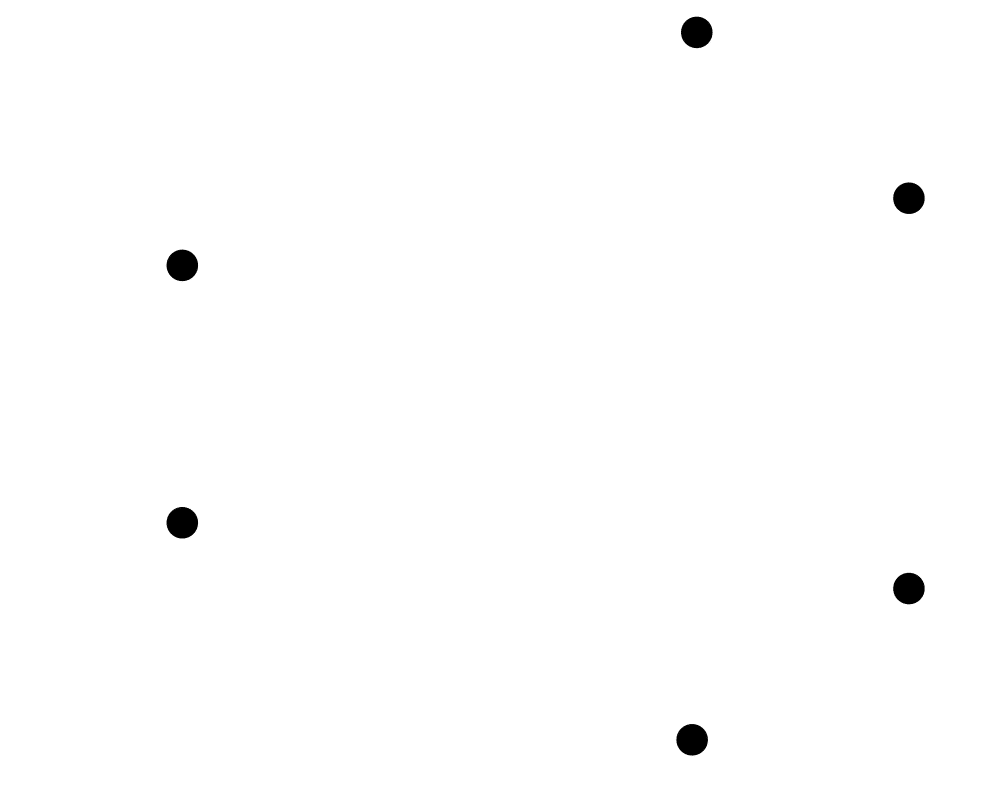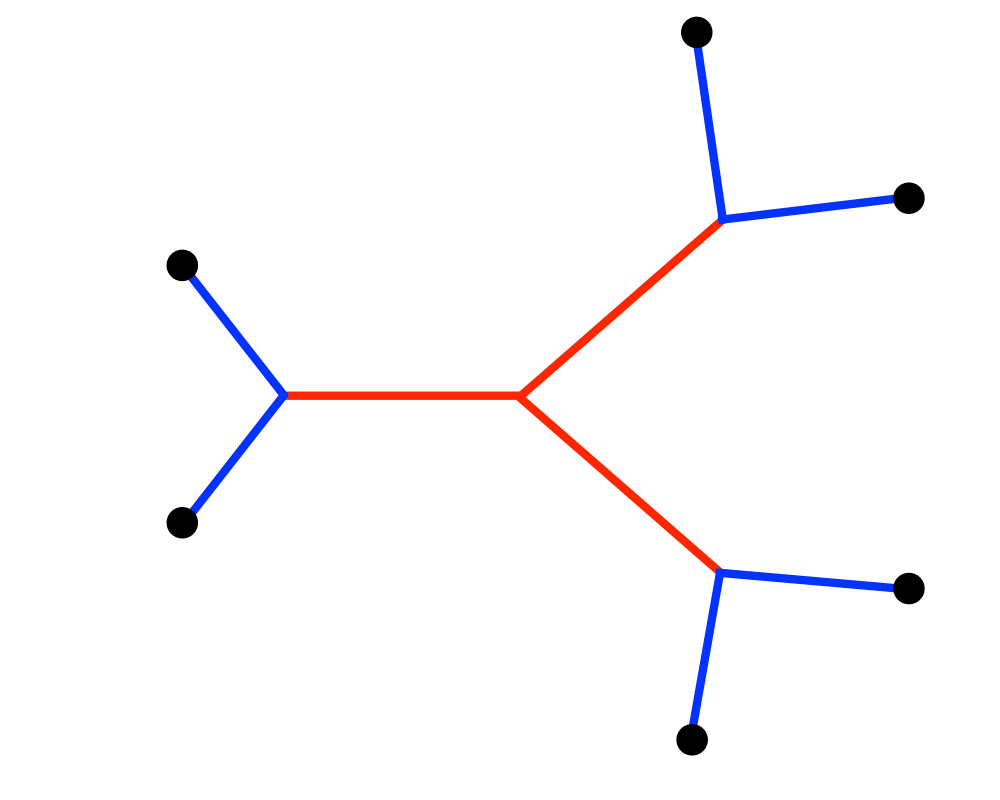# Steiner TreeInputOutput

Input Description: A graph $$G=(V,E)$$. A subset of vertices $$T \in V$$.
Problem: Find the smallest tree connecting all the vertices of $$T$$.

Excerpt from The Algorithm Design Manual: Steiner tree often arises in network design and wiring layout problems. Suppose we are given a set of sites that must be connected by wires as cheaply as possible. The minimum Steiner tree describes the way to connect them using the smallest amount of wire. Analogous problems arise in designing networks of water pipes or heating ducts in buildings. Similar considerations also arise in VLSI circuit layout, where we seek to connect a set of sites to (say) ground under constraints such as material cost, signal propagation time, or reducing capacitance.

The Steiner tree problem is distinguished from the minimum spanning tree problem in that we are permitted to construct or select intermediate connection points to reduce the cost of the tree.

Go To Main Page